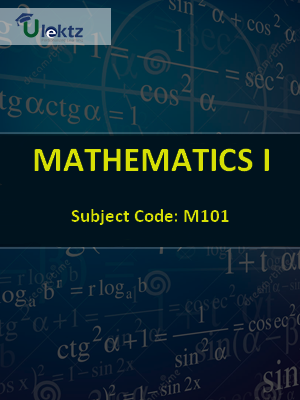•My WalletMy Order
•My Profile
•My Connections
•My Books
•My Videos
•My Tests
•My Calender
•My Messages
•My Shopping Cart
•My Orders
•Account Settings
•Help

# Book Details# MATHEMATICS-I

 Course Code : M101 Author : uLektz University : Maulana Abul Kalam Azad University of Technology Regulation : 2010 Categories : Computer Science Format :ePUB3 (DRM Protected) Type : eBook

FREE

Description :MATHEMATICS-I of M101 covers the latest syllabus prescribed by Maulana Abul Kalam Azad University of Technology for regulation 2010. Author: uLektz, Published by uLektz Learning Solutions Private Limited.

Note : No printed book. Only ebook. Access eBook using uLektz apps for Android, iOS and Windows Desktop PC.

##### Topics
###### MODULE I MATRIX

1.1 Determinant of a square matrix, Minors and Cofactors, Laplaces method of expansion of a determinant, Product of two determinants, Adjoint of a determinant, Jacobis theorem on adjoint determinant

1.2 Singular and non-singular matrices, Adjoint of a matrix, Inverse of a non-singular matrix and its properties, orthogonal matrix and its properties, Trace of a matrix

1.3 Rank of a matrix and its determination using elementary row and column operations

1.4 Solution of simultaneous linear equations by matrix inversion method, Consistency and inconsistency of a system of homogeneous and inhomogeneous linear simultaneous equations

1.5 Eigen values and eigen vectors of a square matrix (of order 2 or 3), Eigen values of APTP, kA, AP-1P

1.6 Cayley-Hamilton theorem and its applications

###### MODULE II SUCCESSIVE DIFFERENTIATION, MEAN VALUE THEOREMS & EXPANSION OF FUNCTIONS

2.1 Successive differentiation: Higher order derivatives of a function of single variable, Leibnitzs theorem (statement only and its application), Problems of the type of recurrence relations in derivatives of different orders and also to find ((yn)0)

2.2 Mean Value Theorems & Expansion of Functions: Rolles theorem and its application, Mean Value theorems  Lagrange & Cauchy and their application

2.3 Taylor’s theorem with Lagrange’s and Cauchy’s form of remainders and its application, Expansions of functions by Taylor’s and Maclaurin’s theorem, Maclaurin’s infinite series expansion of the functions: sin x, cos x ,ex, log(1+x), (a+x)n, n being an integer or a fraction (assuming that the remainder Rn →0 as n → ∞ in each case)

2.4 Reduction formula: Reduction formulae both for indefinite and definite integrals of types, m,n are positive integers

###### MODULE III CALCULUS OF FUNCTIONS OF SEVERAL VARIABLES

3.1 Introduction to functions of several variables with examples, Knowledge of limit and continuity

3.2 Partial derivatives and related problems, Homogeneous functions and Euler’s theorem and related problems upto three variables, Chain rules

3.3 Differentiation of implicit functions

3.4 Total differentials and their related problems, Jacobians up to three variables and related problems

3.5 Maxima, minima and saddle points of functions and related problems

3.6 Concept of line integrals, Double and triple integrals

###### MODULE IV INFINITE SERIES

4.1 Preliminary ideas of sequence, Infinite series and their convergence/divergence, Infinite series of positive terms

4.2 Tests for convergence: Comparison test, Cauchy’s Root test, D’ Alembert’s Ratio test, Raabe’s test (statements and related problems on these tests)

4.3 Alternating series, Leibnitz’s Test (statement, definition) illustrated by simple example, Absolute convergence and Conditional convergence

###### MODULE V VECTOR ALGEBRA AND VECTOR CALCULUS

5.1 Scalar and vector fields–definition and terminologies, Dot and cross products, Scalar and vector triple products and related problems, Equation of straight line, plane and sphere, Vector function of a scalar variable

5.2 Differentiation of a vector function, Scalar and vector point functions, Gradient of a scalar point function, Divergence and curl of a vector point function, Directional derivative, Related problems on these topics

5.3 Green’s theorem, Gauss Divergence Theorem and Stoke’s theorem (Statements and applications)

### Related Books